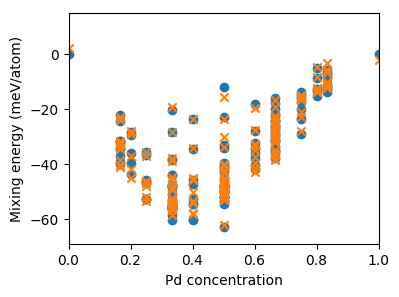# Comparison with target data¶

In this step the performance of the cluster expansion constructed in the previous step will be tested against the target data. After loading the CE from file, we loop over all configurations in the database of reference structure and compile the concentration as well as the target and predicted mixing energies into a dictionary. Note that the latter of the three values is calculated by calling the `predict()` method of the `ClusterExpansion` object with the ```ASE Atoms``` object that represents the present structure as input argument.

```ce = ClusterExpansion.read('mixing_energy.ce')
data = {'concentration': [], 'reference_energy': [], 'predicted_energy': []}
db = connect('reference_data.db')
for row in db.select('natoms<=6'):
data['concentration'].append(row.concentration)
# the factor of 1e3 serves to convert from eV/atom to meV/atom
data['reference_energy'].append(1e3 * row.mixing_energy)
data['predicted_energy'].append(1e3 * ce.predict(row.toatoms()))

```

Once this step has completed the predicted and target mixing energies are plotted as functions of the concentration.

```fig, ax = plt.subplots(figsize=(4, 3))
ax.set_xlabel(r'Pd concentration')
ax.set_ylabel(r'Mixing energy (meV/atom)')
ax.set_xlim([0, 1])
ax.set_ylim([-69, 15])
ax.scatter(data['concentration'], data['reference_energy'],
marker='o', label='reference')
ax.scatter(data['concentration'], data['predicted_energy'],
marker='x', label='CE prediction')
plt.savefig('mixing_energy_comparison.png', bbox_inches='tight')
```

The figure generated by this diagram is shown below.Predicted (orange crosses) and target (blue circles) mixing energies versus concentration for the structures used in the construction of the cluster expansion.¶

## Source code¶

The complete source code is available in `examples/tutorial/2_compare_to_target_data.py`

```# This scripts runs in about 6 seconds on an i7-6700K CPU.

import matplotlib.pyplot as plt
from ase.db import connect
from icet import ClusterExpansion

# step 1: Compile predicted and reference data for plotting
data = {'concentration': [], 'reference_energy': [], 'predicted_energy': []}
db = connect('reference_data.db')
for row in db.select('natoms<=6'):
data['concentration'].append(row.concentration)
# the factor of 1e3 serves to convert from eV/atom to meV/atom
data['reference_energy'].append(1e3 * row.mixing_energy)
data['predicted_energy'].append(1e3 * ce.predict(row.toatoms()))

# step 2: Plot results
fig, ax = plt.subplots(figsize=(4, 3))
ax.set_xlabel(r'Pd concentration')
ax.set_ylabel(r'Mixing energy (meV/atom)')
ax.set_xlim([0, 1])
ax.set_ylim([-69, 15])
ax.scatter(data['concentration'], data['reference_energy'],
marker='o', label='reference')
ax.scatter(data['concentration'], data['predicted_energy'],
marker='x', label='CE prediction')
plt.savefig('mixing_energy_comparison.png', bbox_inches='tight')
```